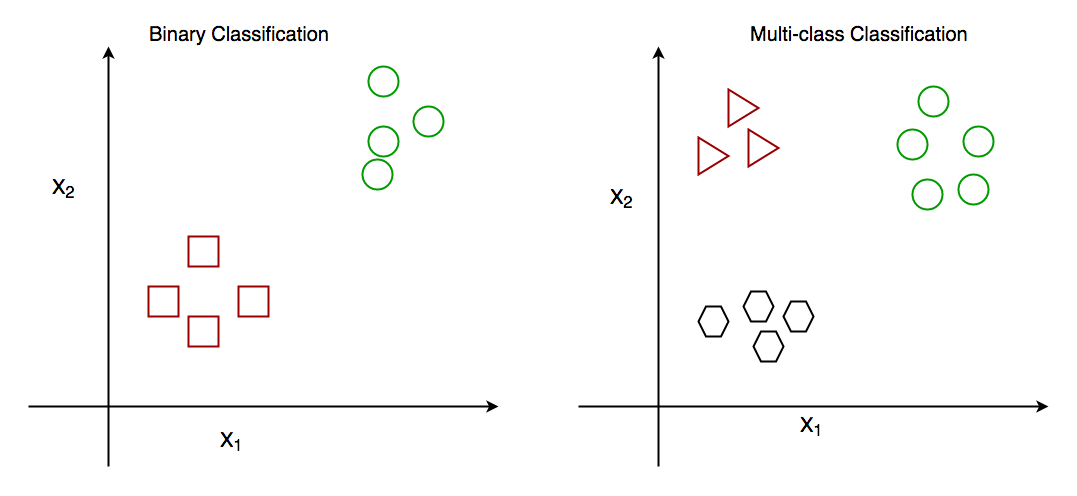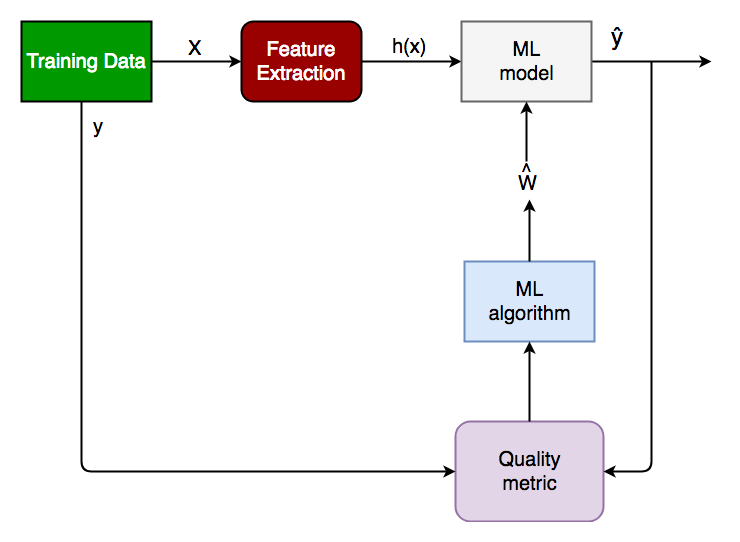Skip to content
Related Articles
Getting started with Classification
• Difficulty Level : Easy
• Last Updated : 09 Feb, 2018

Introduction

As the name suggests, Classification is the task of “classifying things” into sub-categories.But, by a machine! If that doesn’t sound like much, imagine your computer being able to differentiate between you and a stranger. Between a potato and a tomato. Between an A grade and a F- .

Yeah. It sounds interesting now!

In Machine Learning and Statistics, Classification is the problem of identifying to which of a set of categories (sub populations), a new observation belongs to, on the basis of a training set of data containing observations and whose categories membership is known.

Types of Classification

Classification is of two types:

• Binary Classification : When we have to categorize given data into 2 distinct classes. Example – On the basis of given health conditions of a person, we have to determine whether the person has a certain disease or not.
• Multiclass Classification : The number of classes is more than 2. For Example – On the basis of data about different species of flowers, we have to determine which specie does our observation belong to.Fig : Binary and Multiclass Classification. Here x1 and x2 are our variables upon which the class is predicted.

How does classification works?

Suppose we have to predict whether a given patient has a certain disease or not, on the basis of 3 variables, called features.

Which means there are two possible outcomes:

1. The patient has the said disease. Basically a result labelled “Yes” or “True”.
2. The patient is disease free. A result labelled “No” or “False”.

This is a binary classification problem.

We have a set of observations called training data set, which comprises of sample data with actual classification results. We train a model, called Classifier on this data set, and use that model to predict whether a certain patient will have the disease or not.

The outcome, thus now depends upon :

1. How well these features are able to “map” to the outcome.
2. The quality of our data set. By quality I refer to statistical and Mathematical qualities.
3. How well our Classifier generalizes this relationship between the features and the outcome.
4. The values of the x1 and x2.

Following is the generalized block diagram of the classification task.Generalized Classification Block Diagram.

1. X : pre-classified data, in the form of a N*M matrix. N is the no. of observations and M is the number of features
2. y : An N-d vector corresponding to predicted classes for each of the N observations.
3. Feature Extraction : Extracting valuable information from input X using a series of transforms.
4. ML Model : The “Classifier” we’ll train.
5. y’ : Labels predicted by the Classifier.
6. Quality Metric : Metric used for measuring the performance of the model.
7. ML Algorithm : The algorithm that is used to update weights w’, which update the model and “learns” iteratively.

Types of Classifiers (algorithms)

There are various types of classifiers. Some of them are :

• Linear Classifiers : Logistic Regression
• Tree Based Classifiers : Decision Tree Classifier
• Support Vector Machines
• Artificial Neural Networks
• Bayesian Regression
• Gaussian Naive Bayes Classifiers
• Stochastic Gradient Descent (SGD) Classifier
• Ensemble Methods : Random Forests, AdaBoost, Bagging Classifier, Voting Classifier, ExtraTrees Classifier

Detailed description of these methodologies is beyond an article!

Practical Applications of Classification

1. Google’s self driving car uses deep learning enabled classification techniques which enables it to detect and classify obstacles.
2. Spam E-mail filtering is one of the most widespread and well recognized uses of Classification techniques.
3. Detecting Health Problems, Facial Recognition, Speech Recognition, Object Detection, Sentiment Analysis all use Classification at their core.

Implementation

Let’s get a hands on experience at how Classification works.We are going to study about various Classifiers and see a rather simple analytical comparison of their performance on a well known, standard data set, the Iris data set.

### Requirements for running the given script

1. Python 2.7
2. Scipy and Numpy
3. Matplotlib for data visualization
4. Pandas for data i/o
5. Scikit-learn Provides all the classifiers

Python Implementation- Github link to the Project

Conclusion

Classification is a very vast field of study. Even though it comprises of a small part of Machine Learning as a whole, it is one of the most important ones.

That’s all for now. In the next article, we will see how Classification works in practice and get our hands dirty with Python Code.

This article is contributed by Sarthak Yadav. If you like GeeksforGeeks and would like to contribute, you can also write an article and mail your article to contribute@geeksforgeeks.org. See your article appearing on the GeeksforGeeks main page and help other Geeks.

Please write comments if you find anything incorrect, or you want to share more information about the topic discussed aboveMy Personal Notes arrow_drop_up